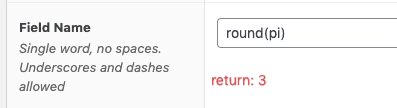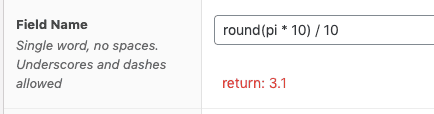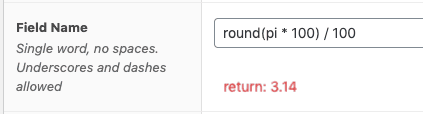# Rounding functions

All functions in Calculated Fields for ACF take exactly one argument. This works out well in most situations, but for rounding functions, this might become a limitation. For instance, when the resulting number represents a price, it might be convenient to round to a precision of two decimals.

The best way to overcome this is to use a little math trick. First, multiply the function argument with a multiple of 10 and then divide the result with the same multiplier of 10. Examples:

Round to the nearest integer:Round with one decimal precision:Round with two decimals precision:Note that this trick works well with the other rounding functions, `ceil()` and `floor()`